# Optimization Algorithms for Deep Learning

Deep learning is a highly iterative process. We have to try out various permutations of the hyperparameters to figure out which combination works best. Therefore it is crucial that our deep learning model trains in a shorter time without penalizing the cost. In this post, I’ll explain the math behind the most commonly used optimization algorithms used in deep learning.

# Optimization Algorithm

Given an algorithm f(x), an optimization algorithm help in either minimizing or maximizing the value of f(x). In the context of deep learning, we use optimization algorithms to train the neural network by optimizing the cost function J. The cost function is defined as:

The value of cost function J is the mean of the loss L between the predicted value y’ and actual value y. The value y’ is obtained during the forward propagation step and makes use of the Weights W and biases b of the network. With the help of optimization algorithms, we minimize the value of Cost Function J by updating the values of the trainable parameters W and b.

# Gradient Descent

The Weight Matrix W is initialized randomly. We use gradient descent to minimize the Cost Function J and obtain the optimal Weight Matrix W and Bias b. Gradient descent is a first-order iterative optimization algorithm for finding the minimum of a function. We apply gradient descent to the Cost Function J to minimize the cost. Mathematically it can be defined as:

The first equation represents the change in Weight Matrix W, whereas the second equation represents the change in Bias b. The change in the values is determined by learning rate alpha and the derivatives of the cost J with respect to the Weight Matrix W and Bias b. We repeat the updation of Wand b until the Cost Function J has been minimized. Now lets us understand how Gradient Descent works with the help of the following graph:

Case 1. Let us assume that W was initialized with values less than the values at which it achieves a global minimum. The slope at that point, i.e., the partial derivative of J with respect to W is negative, and hence, the weight value increases according to the Gradient Descent’s equation.

Case 2. Let us assume that W was initialized with values more than the values at which it achieves a global minimum. The slope at that point, i.e., the partial derivative of J with respect to W is positive, and hence, the weight value decreases according to the Gradient Descent’s equation.

Accordingly, both W and b achieve their optimal value, and the value of cost function J is minimized.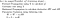Given above, is the basic strategy when we use gradient descent as our optimization algorithm.

# Mini-Batch Gradient Descent

One of the disadvantages of gradient descent is that it begins the Parameter updation only after it goes through the full training data. This poses a challenge when the training data is too big to fit in the computer memory. Mini-Batch gradient descent is a clever workaround that tackles the above problems of Gradient Descent

In Mini-Batch gradient descent, we distribute the whole training data in small mini-batches of sizes 16,32,64, and so on depending on the use case. We then use these mini-batches to train the network iteratively. The use of mini-batch has two advantages:

1. Training starts as soon as we traverse over the first mini-batch, i.e., from the first few training examples.
2. We can train a neural network even when we have a large amount of training data that doesn’t fit in the memory.

The batch_size now becomes a new hyperparameter for our model.

1. When the batch_size = number of training examples, it is called as Batch gradient descent. It faces the problem of beginning learning only after traversing the whole dataset.
2. When the batch_size = 1, it is called as Stochastic Gradient Descent. It does not make full use of vectorization, and the training becomes very slow.
3. Therefore, the common choice is 64 or 128 or 256 or 512. However, it depends on the use case and the system memory, i.e., we should ensure that a single mini-batch should be able to fit in the system memory.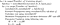Given above, is the basic strategy when we use mini-batch gradient descent as our optimization algorithm.

# Momentum

Gradient Descent with momentum or just Momentum is an advanced optimization algorithm that speeds up the optimization of the cost function J. It makes use of the moving average to update the trainable parameters of the neural network.

Moving average is the average calculated over n successive values rather than the whole set of values. Mathematically, it is denoted as:

Here, A[i] represents the moving average at the i data point for the value X[i]. The parameter β controls the value n over which average is calculated. For example, if β=0.9, the moving average considers 10 successive values to calculate the average; if β=0.99, the moving average considers 100 consecutive values to calculate the average. In general, the value n can be approximated by the following formula:

The figure below shows how moving average works. As the value of β increases, n increases, and the plot moves towards the right, because initially, the values are increasing. Whereas, when β decreases, n decreases, and the scenario correctly models X. Therefore it is essential to figure out the appropriate value of β to get a reasonably good moving average. It is observed that β=0.9 works well in most cases.

Now, that we know what moving average is, let us try to understand how it is used in the Momentum Algorithm. While training a neural network, the goal is to optimize the cost function J and minimize its value. A traditional gradient descent optimizer follows the blue path, whereas the momentum optimizer follows the green path to reach the minimum (Red) point.

The path followed by Gradient Descent takes too many steps as compared to Momentum. This is because the Gradient Descent oscillates too much in the y-axis and moves very less in the x-axis, i.e., toward the minimum. A right solution would be to reduce oscillations by dampening out the motion in the y-axis. This is where the moving average comes into play.

If we observe the blue path, we see that the movement in the y-axis is a series of positive and negative changes. We apply the weighted average to almost zero out the movement and therefore, the oscillations in the y-axis. A similar intuition goes for the movement in the x-axis. This reduces the oscillations in the path, and eventually, the neural network reaches the minimum in a shorter duration along with less number of training iterations. To apply this, we introduce two new variables VdW and Vdb to keep track of the weighted average of the derivatives of weight dW and bias db.

It is worthy to note that we can use the Mini Batch approach along with Moment optimizer as only the Parameter Updation methodology changes.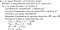Given above, is the basic strategy when we use Momentum as our optimization algorithm.

# RMS Prop

RMS Prop stands for Root-Mean-Square Propagation. Similar to momentum, it is a technique to dampen out the motion in the y-axis. Let out try to understand how it works with the help of our previous example. For better understanding, let us denote the Y-axis as the bias b and the X-axis as the Weight W.

The intuition is that we when divide a large number by another number, the result becomes small. In our case, the first large number is db and the second large number that we use is the weighted average of db². We introduce two new variables Sdb and SdW, to keep track of the weighted average of db² and dW². The division of db and Sdb results in a smaller value which dampens out the movement in the y-axis. The is introduced to avoid the division by 0 error. A similar intuition goes for updation of W, i.e., the value in X-axis.

It is worthy to note that here we have considered Y-axis as bias b and X-axis as weight W for better understanding and to visualize how the parameters are updated. We can damp out any such oscillation either caused by any bias b(b1, b2, …, bn) or weight W(W1, W2, …, Wn) or both in a similar manner. And again, we can still use the Mini Batch approach along with RMS optimizer as only the Parameter Updation methodology changes.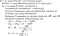Given above, is the basic strategy when we use RMS prop as our optimization algorithm.

## AdaM

AdaM stands for Adaptive Momentum. It combines the Momentum and RMS prop in a single approach making AdaM a very powerful and fast optimizer. Also, we use error correction to solve the cold start problem in weighted average calculation, i.e., the first few values of weighted average are too far fro their real values. The V values incorporate the logic from Momentum, whereas S values incorporate the logic from RMS prop.

It is worthy to note that we use 2 different values of β during the calculations. β1 is used for calculations relevant to Momentum whereas β2 is used for calculations relevant to RMS prop. And again, we can still use the Mini Batch approach along with AdaM optimizer as only the Parameter Updation methodology changes.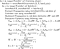Given above, is the basic strategy when we use AdaM as our optimization algorithm.

# Performance Comparison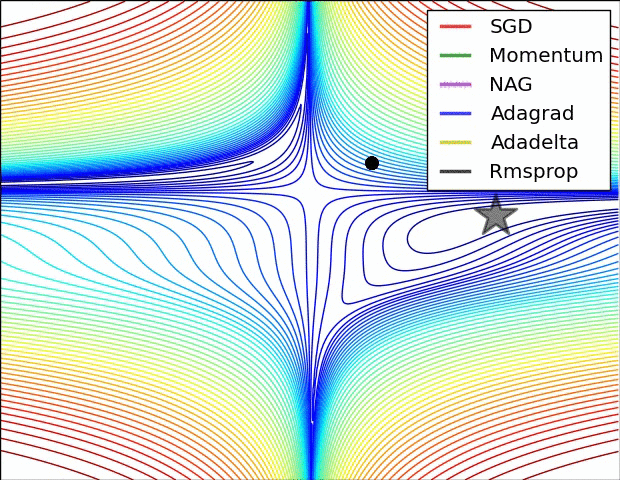Contours of a loss surface and time evolution of different optimization algorithms

# References

I want to thank the readers for reading the story. If you have any questions or doubts, feel free to ask them in the comments section below. I’ll be more than happy to answer them and help you out. If you like the story, please follow me to get regular updates when I publish a new story. I welcome any suggestions that will improve my stories.

Written by

## Analytics Vidhya

#### Analytics Vidhya is a community of Analytics and Data Science professionals. We are building the next-gen data science ecosystem https://www.analyticsvidhya.com

Welcome to a place where words matter. On Medium, smart voices and original ideas take center stage - with no ads in sight. Watch
Follow all the topics you care about, and we’ll deliver the best stories for you to your homepage and inbox. Explore
Get unlimited access to the best stories on Medium — and support writers while you’re at it. Just \$5/month. Upgrade